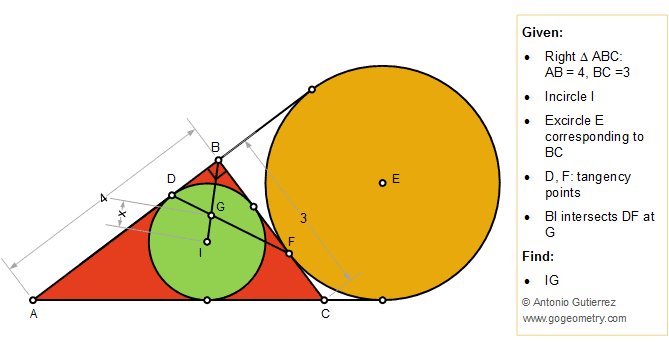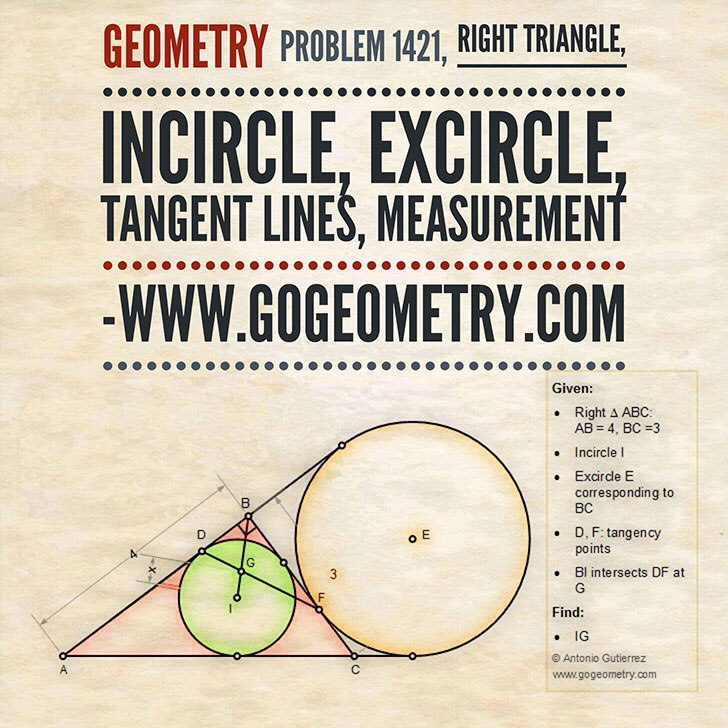Geometry Problem 1421: Right Triangle, Incircle, Excircle, Tangent Lines, Measurement

The figure below shows a right triangle ABC (angle B = 90 degree) with AB = 4 and BC = 3. The incircle I is tangent to AB at D. The excircle E corresponding to BC is tangent to BC at F. BI intersects DF at G. Find the measure of IG.Geometric Art of Problem 1421: Sketching, Typography, iPad Apps